|

# 毛胚屋美丽变身记 215平新古典大户型装修技巧

毛坯房总会有许多值得开发的地方，能够发挥的空间最大。本套案例中，设计师从毛胚屋格局开始，首先延续玄关线条，引日光明亮入门动线，从端景墙延伸的壁纸，串联客餐厅空间形成有效整体，并巧妙利用茶镜与线板的切割，打造井然有序的大宅空间。

案例一：215平新古典毛胚屋

主要建材：线板、大理石、壁纸、茶镜、茶玻

大宅的横向串联至后方改以穿透手法设计，喷纱于拉门上，丰富望向厨房的视觉层次，书房的清玻隔间也拉阔空间宽度，在奢美风家具镜面拉扣的光泽映掩中，铺叙豪宅尺度的清朗奢华。玄关设计

重点设计：玄关空间多用途

编辑点评：清香袅袅处，顶级名牌logo水刀切割拼贴于玄关地坪上，看似无交集的禅净与时尚在日光倾泻下，有了完美的链结表情。客厅设计

重点设计：延伸线条

编辑点评：从端景墙延伸的壁纸语汇向内串联客餐厅，巧妙利用茶镜与线板的切割形塑井然的大宅层次。客厅设计

重点设计：电视墙

编辑点评：设计师将视听设备整合于茶玻门片后方，完整的立面混搭茶玻与柜体线条，也进而美化客卫浴墙面设计。客厅设计

重点设计：大容量收纳

编辑点评：细浅的线条内蕴涵真实完整的大容量收纳，满足居住的需求。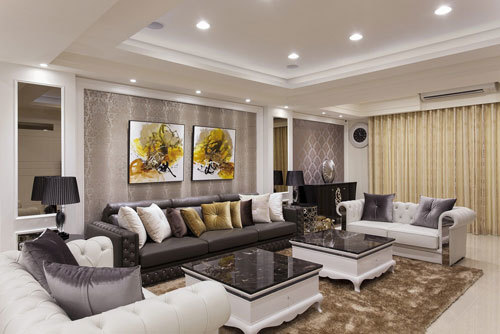客厅设计

重点设计：客厅沙发背景墙

编辑点评：石材搭配烤漆的双茶几，与水钻拉扣新古典线条沙发，从家具软装烘托奢美馨韵的家居空间。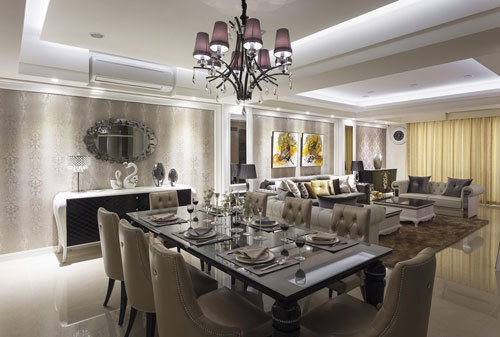餐厅设计

重点设计：餐厅吊顶

编辑点评：春雨设计以造型矮餐柜取代传统高柜设计，与威尼斯镜共塑宴会厅的华美气度。

X餐厅设计

重点设计：背景门片

编辑点评：大宅的横向串联至后方改以穿透手法续写，喷纱于拉门上的落英缤纷了望向厨房的视觉层次。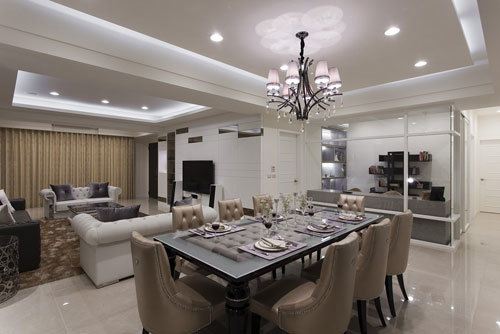餐厅设计

重点设计：穿透设计手法

编辑点评：书房的清玻隔间也拉阔空间宽度，串联起餐厅客厅的空间。书房设计

重点设计：书房收纳

编辑点评：木作打造的书桌预先规划上掀盖式线路插座，保持桌面与穿透视野的干净齐整。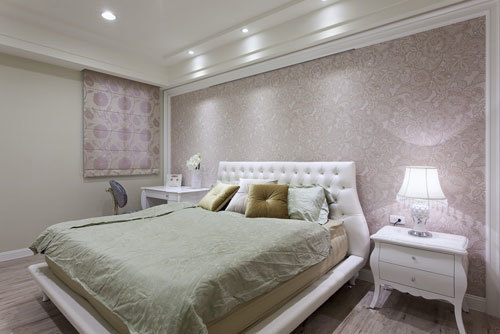卧室设计

重点设计：主卧房床头背景墙

编辑点评：粉色系壁纸与白色床头板柔美主卧房氛围。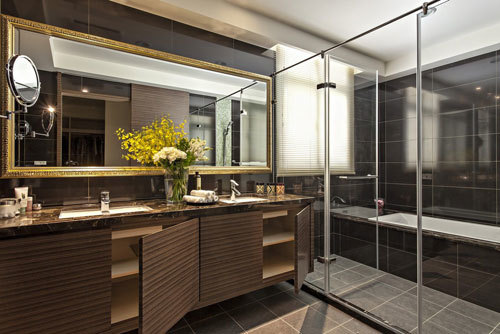卫浴设计

重点设计：主卫浴干湿分离

编辑点评：大理石台面与艺术画框镜面提升卫浴质感，下方浴柜更有实质的收纳机能。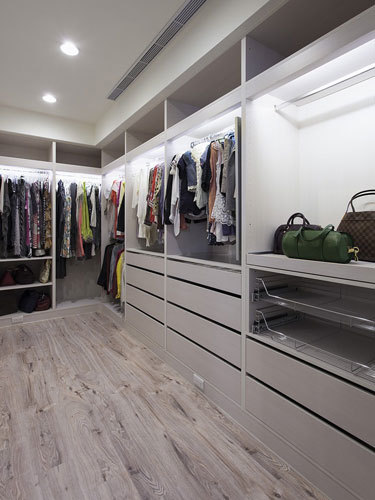衣帽间设计

重点设计：更衣室强收纳

编辑点评：春雨设计考量屋主的服饰配件，打造温馨机能的更衣空间。

X

案例二：241平美式毛胚屋

主要建材：大理石、壁纸、艺术画框、半抛磁砖、木纹砖

月牙弯般的乳白象牙呼应繁复拼花地坪，烛台造型水晶灯从棚顶悬垂而下，入内视觉轻易就被掳获，如艺品般的经典家具，巧妙安排在崭新的设计线条中，在新旧融合外，更具有传世艺文价值。

由地板分区，餐厅格栅门片的后方是家人主要的活动空间，文化石围塑的空间内，三米长的木作中岛是家人共享天伦早午餐，也是夫妻啜饮品酒的lounge吧台，在修饰梁体而增设的木作格栅天花映衬下，晕染一室温柔地美式乡村情调。玄关设计

重点设计：玄关地板分区

编辑点评：月牙弯般的乳白象牙呼应繁复拼花地坪，烛台造型水晶灯从棚顶悬垂而下，气宇不凡的大宅气势从玄关轻启。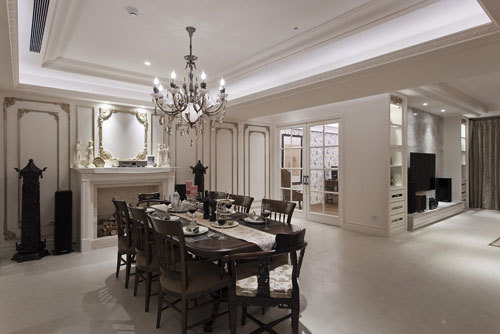客厅设计

重点设计：描金烘托奢华空间

编辑点评：入内视觉轻易就被金漆描边的细致雕花线板掳获，与屋主的艺品收藏完美融会出美式宅邸的文艺沙龙风貌。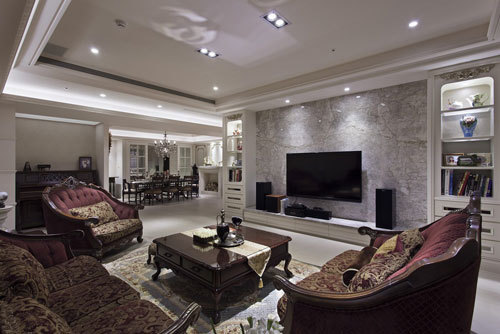客厅设计

重点设计：电视墙

编辑点评：大理石线条呼应两侧书柜设计，达到视觉上的美好平衡。客厅设计

重点设计：客厅新旧结合

编辑点评：如艺品般的经典家具，巧妙安排在崭新的设计线条中，在新旧融合外，更具有传世艺文价值。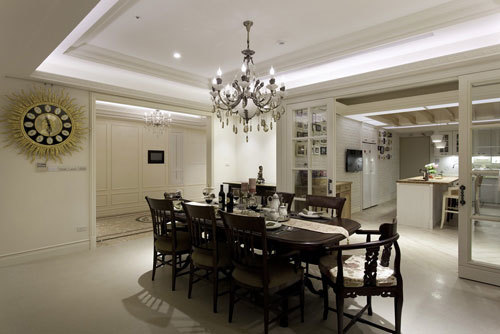餐厅设计

重点设计：餐厅分区

编辑点评：由地板的分野，越过餐厅半抛磁砖后方，木纹砖地板点出厨房区的温暖乡村氛围。厨房设计

重点设计：厨房照片墙

编辑点评：文化石围塑的空间内在修饰梁体而增设的木作格栅天花映衬下，晕染一室温柔地美式乡村情调。 X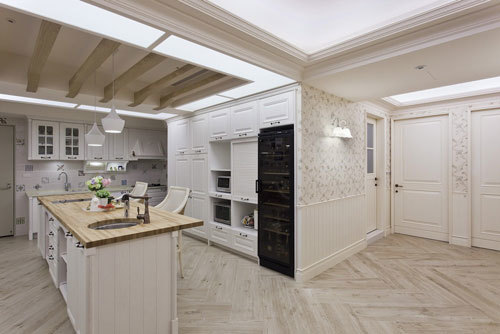厨房设计

重点设计：规划生活动线

编辑点评：厨房规划为家人主要的活动场所，三米长的木作中岛是家人共享天伦早午餐，也是夫妻啜饮品酒的lounge吧台。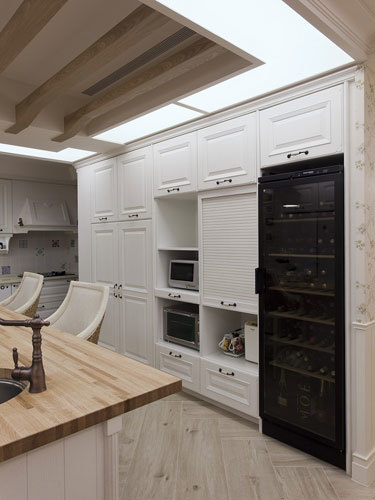厨房设计

重点设计：收纳设计

编辑点评：每一个线板线条内皆为特定基能规画收纳空间。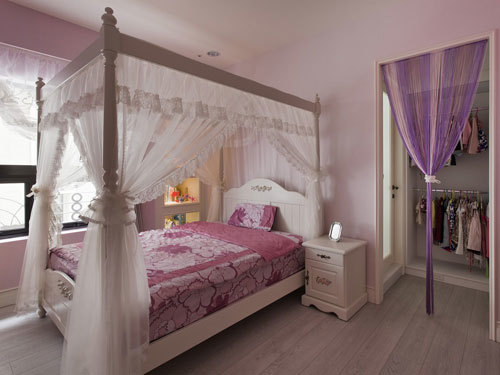儿童房设计

重点设计：女孩房更衣室

编辑点评：粉红柔美的女孩房还有专属更衣室计画。卧室设计

重点设计：主卧房床头背景墙

编辑点评：立面轻覆简约线板与熏衣草色漆面浪漫呈现，可调光地木百叶窗更画龙点睛出美式氛围。衣帽间设计

重点设计：更衣室规划

编辑点评：除了包包、衣饰展示收纳机能外，还有简单盥洗及阅读设计，开创更衣室全新格局。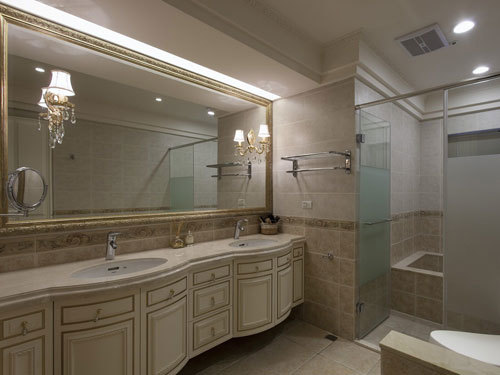卫浴设计

重点设计：主卫浴画框氛围设计

编辑点评：设计师运用进口砖与艺术画框，营造星级饭店等级的卫浴空间。

`声明：本文由入驻焦点开放平台的作者撰写，除焦点官方账号外，观点仅代表作者本人，不代表焦点立场错误信息举报电话： 400-099-0099，邮箱：jubao@vip.sohu.com，或点此进行意见反馈，或点此进行举报投诉。`A B C D E F G H J K L M N P Q R S T W X Y Z
A - B - C - D - E
• A
• 鞍山
• 安庆
• 安阳
• 安顺
• 安康
• 澳门
• B
• 北京
• 保定
• 包头
• 巴彦淖尔
• 本溪
• 蚌埠
• 亳州
• 滨州
• 北海
• 百色
• 巴中
• 毕节
• 保山
• 宝鸡
• 白银
• 巴州
• C
• 承德
• 沧州
• 长治
• 赤峰
• 朝阳
• 长春
• 常州
• 滁州
• 池州
• 长沙
• 常德
• 郴州
• 潮州
• 崇左
• 重庆
• 成都
• 楚雄
• 昌都
• 慈溪
• 常熟
• D
• 大同
• 大连
• 丹东
• 大庆
• 东营
• 德州
• 东莞
• 德阳
• 达州
• 大理
• 德宏
• 定西
• 儋州
• 东平
• E
• 鄂尔多斯
• 鄂州
• 恩施
F - G - H - I - J
• F
• 抚顺
• 阜新
• 阜阳
• 福州
• 抚州
• 佛山
• 防城港
• G
• 赣州
• 广州
• 桂林
• 贵港
• 广元
• 广安
• 贵阳
• 固原
• H
• 邯郸
• 衡水
• 呼和浩特
• 呼伦贝尔
• 葫芦岛
• 哈尔滨
• 黑河
• 淮安
• 杭州
• 湖州
• 合肥
• 淮南
• 淮北
• 黄山
• 菏泽
• 鹤壁
• 黄石
• 黄冈
• 衡阳
• 怀化
• 惠州
• 河源
• 贺州
• 河池
• 海口
• 红河
• 汉中
• 海东
• I
• J
• 晋中
• 锦州
• 吉林
• 鸡西
• 佳木斯
• 嘉兴
• 金华
• 景德镇
• 九江
• 吉安
• 济南
• 济宁
• 焦作
• 荆门
• 荆州
• 江门
• 揭阳
• 金昌
• 酒泉
• 嘉峪关
K - L - M - N - P
• K
• 开封
• 昆明
• 昆山
• L
• 廊坊
• 临汾
• 辽阳
• 连云港
• 丽水
• 六安
• 龙岩
• 莱芜
• 临沂
• 聊城
• 洛阳
• 漯河
• 娄底
• 柳州
• 来宾
• 泸州
• 乐山
• 六盘水
• 丽江
• 临沧
• 拉萨
• 林芝
• 兰州
• 陇南
• M
• 牡丹江
• 马鞍山
• 茂名
• 梅州
• 绵阳
• 眉山
• N
• 南京
• 南通
• 宁波
• 南平
• 宁德
• 南昌
• 南阳
• 南宁
• 内江
• 南充
• P
• 盘锦
• 莆田
• 平顶山
• 濮阳
• 攀枝花
• 普洱
• 平凉
Q - R - S - T - W
• Q
• 秦皇岛
• 齐齐哈尔
• 衢州
• 泉州
• 青岛
• 清远
• 钦州
• 黔南
• 曲靖
• 庆阳
• R
• 日照
• 日喀则
• S
• 石家庄
• 沈阳
• 双鸭山
• 绥化
• 上海
• 苏州
• 宿迁
• 绍兴
• 宿州
• 三明
• 上饶
• 三门峡
• 商丘
• 十堰
• 随州
• 邵阳
• 韶关
• 深圳
• 汕头
• 汕尾
• 三亚
• 三沙
• 遂宁
• 山南
• 商洛
• 石嘴山
• T
• 天津
• 唐山
• 太原
• 通辽
• 铁岭
• 泰州
• 台州
• 铜陵
• 泰安
• 铜仁
• 铜川
• 天水
• 天门
• W
• 乌海
• 乌兰察布
• 无锡
• 温州
• 芜湖
• 潍坊
• 威海
• 武汉
• 梧州
• 渭南
• 武威
• 吴忠
• 乌鲁木齐
X - Y - Z
• X
• 邢台
• 徐州
• 宣城
• 厦门
• 新乡
• 许昌
• 信阳
• 襄阳
• 孝感
• 咸宁
• 湘潭
• 湘西
• 西双版纳
• 西安
• 咸阳
• 西宁
• 仙桃
• 西昌
• Y
• 运城
• 营口
• 盐城
• 扬州
• 鹰潭
• 宜春
• 烟台
• 宜昌
• 岳阳
• 益阳
• 永州
• 阳江
• 云浮
• 玉林
• 宜宾
• 雅安
• 玉溪
• 延安
• 榆林
• 银川
• Z
• 张家口
• 镇江
• 舟山
• 漳州
• 淄博
• 枣庄
• 郑州
• 周口
• 驻马店
• 株洲
• 张家界
• 珠海
• 湛江
• 肇庆
• 中山
• 自贡
• 资阳
• 遵义
• 昭通
• 张掖
• 中卫

1室1厅1厨1卫1阳台

1
2
3
4
5

0
1
2

1

1

0
1
2
3报名成功，资料已提交审核A B C D E F G H J K L M N P Q R S T W X Y Z
A - B - C - D - E
• A
• 鞍山
• 安庆
• 安阳
• 安顺
• 安康
• 澳门
• B
• 北京
• 保定
• 包头
• 巴彦淖尔
• 本溪
• 蚌埠
• 亳州
• 滨州
• 北海
• 百色
• 巴中
• 毕节
• 保山
• 宝鸡
• 白银
• 巴州
• C
• 承德
• 沧州
• 长治
• 赤峰
• 朝阳
• 长春
• 常州
• 滁州
• 池州
• 长沙
• 常德
• 郴州
• 潮州
• 崇左
• 重庆
• 成都
• 楚雄
• 昌都
• 慈溪
• 常熟
• D
• 大同
• 大连
• 丹东
• 大庆
• 东营
• 德州
• 东莞
• 德阳
• 达州
• 大理
• 德宏
• 定西
• 儋州
• 东平
• E
• 鄂尔多斯
• 鄂州
• 恩施
F - G - H - I - J
• F
• 抚顺
• 阜新
• 阜阳
• 福州
• 抚州
• 佛山
• 防城港
• G
• 赣州
• 广州
• 桂林
• 贵港
• 广元
• 广安
• 贵阳
• 固原
• H
• 邯郸
• 衡水
• 呼和浩特
• 呼伦贝尔
• 葫芦岛
• 哈尔滨
• 黑河
• 淮安
• 杭州
• 湖州
• 合肥
• 淮南
• 淮北
• 黄山
• 菏泽
• 鹤壁
• 黄石
• 黄冈
• 衡阳
• 怀化
• 惠州
• 河源
• 贺州
• 河池
• 海口
• 红河
• 汉中
• 海东
• I
• J
• 晋中
• 锦州
• 吉林
• 鸡西
• 佳木斯
• 嘉兴
• 金华
• 景德镇
• 九江
• 吉安
• 济南
• 济宁
• 焦作
• 荆门
• 荆州
• 江门
• 揭阳
• 金昌
• 酒泉
• 嘉峪关
K - L - M - N - P
• K
• 开封
• 昆明
• 昆山
• L
• 廊坊
• 临汾
• 辽阳
• 连云港
• 丽水
• 六安
• 龙岩
• 莱芜
• 临沂
• 聊城
• 洛阳
• 漯河
• 娄底
• 柳州
• 来宾
• 泸州
• 乐山
• 六盘水
• 丽江
• 临沧
• 拉萨
• 林芝
• 兰州
• 陇南
• M
• 牡丹江
• 马鞍山
• 茂名
• 梅州
• 绵阳
• 眉山
• N
• 南京
• 南通
• 宁波
• 南平
• 宁德
• 南昌
• 南阳
• 南宁
• 内江
• 南充
• P
• 盘锦
• 莆田
• 平顶山
• 濮阳
• 攀枝花
• 普洱
• 平凉
Q - R - S - T - W
• Q
• 秦皇岛
• 齐齐哈尔
• 衢州
• 泉州
• 青岛
• 清远
• 钦州
• 黔南
• 曲靖
• 庆阳
• R
• 日照
• 日喀则
• S
• 石家庄
• 沈阳
• 双鸭山
• 绥化
• 上海
• 苏州
• 宿迁
• 绍兴
• 宿州
• 三明
• 上饶
• 三门峡
• 商丘
• 十堰
• 随州
• 邵阳
• 韶关
• 深圳
• 汕头
• 汕尾
• 三亚
• 三沙
• 遂宁
• 山南
• 商洛
• 石嘴山
• T
• 天津
• 唐山
• 太原
• 通辽
• 铁岭
• 泰州
• 台州
• 铜陵
• 泰安
• 铜仁
• 铜川
• 天水
• 天门
• W
• 乌海
• 乌兰察布
• 无锡
• 温州
• 芜湖
• 潍坊
• 威海
• 武汉
• 梧州
• 渭南
• 武威
• 吴忠
• 乌鲁木齐
X - Y - Z
• X
• 邢台
• 徐州
• 宣城
• 厦门
• 新乡
• 许昌
• 信阳
• 襄阳
• 孝感
• 咸宁
• 湘潭
• 湘西
• 西双版纳
• 西安
• 咸阳
• 西宁
• 仙桃
• 西昌
• Y
• 运城
• 营口
• 盐城
• 扬州
• 鹰潭
• 宜春
• 烟台
• 宜昌
• 岳阳
• 益阳
• 永州
• 阳江
• 云浮
• 玉林
• 宜宾
• 雅安
• 玉溪
• 延安
• 榆林
• 银川
• Z
• 张家口
• 镇江
• 舟山
• 漳州
• 淄博
• 枣庄
• 郑州
• 周口
• 驻马店
• 株洲
• 张家界
• 珠海
• 湛江
• 肇庆
• 中山
• 自贡
• 资阳
• 遵义
• 昭通
• 张掖
• 中卫• 手机• 分享
• 设计
免费设计
• 计算器
装修计算器
• 入驻
合作入驻
• 联系
联系我们
• 置顶
返回顶部